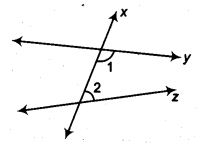NCERT Solutions for Class 9 Maths Chapter 3 Introduction to Euclid’s Geometry Ex 3.2 are part of NCERT Solutions for Class 9 Maths. Here we have given NCERT Solutions for Class 9 Maths Chapter 3 Introduction to Euclid’s Geometry Ex 3.2.

 Board CBSE Textbook NCERT Class Class 9 Subject Maths Chapter Chapter 3 Chapter Name Introduction to Euclid’s Geometry Exercise Ex 3.2 Number of Questions Solved 2 Category NCERT Solutions

## NCERT Solutions for Class 9 Maths Chapter 3 Introduction to Euclid’s Geometry Ex 3.2

Question 1.
How would you rewrite Euclid’s fifth postulate so that it would be easier to understand?
Solution:
Two distinct intersecting lines cannot be parallel to the same line.

Question 2.
Does Euclid’s fifth postulate imply the existence of parallel lines? Explain.
Solution:
Yes.
According to Euclid’s fifth postulate when line x falls on line y and z such that ∠1+ ∠2< 180°. Then, line y and line z on producing further will meet in the side of ∠1 arid ∠2 which is less than 180°.We find that the lines which are not according to Euclid’s fifth postulate. i.e., ∠1 + ∠2 = 180°, do not intersect.

We hope the NCERT Solutions for Class 9 Maths Chapter 3 Introduction to Euclid’s Geometry Ex 3.2 help you. If you have any query regarding NCERT Solutions for Class 9 Maths Chapter 3 Introduction to Euclid’s Geometry Ex 3.2, drop a comment below and we will get back to you at the earliest.